# Minimal Inequivalent Square Tilings

A dataset of images and constraints for the minimal inequivalent square tilings, along with the allowed tiles that generate the tiling

Originator: Stephen Wolfram

This dataset was presented in its original form on pages 214 and 215 of Stephen Wolfram's "A New Kind of Science".

The function for generating a tiling from a constraint is also provided.

## Examples

### Basic Examples

Retrieve the resource:

 In:=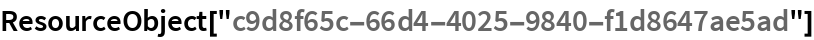Out=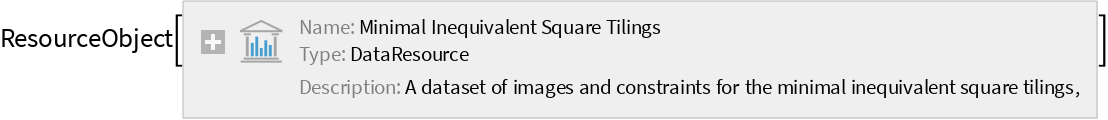Retrieve the default content:

 In:=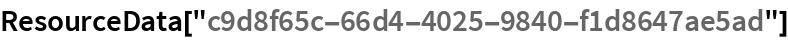Out=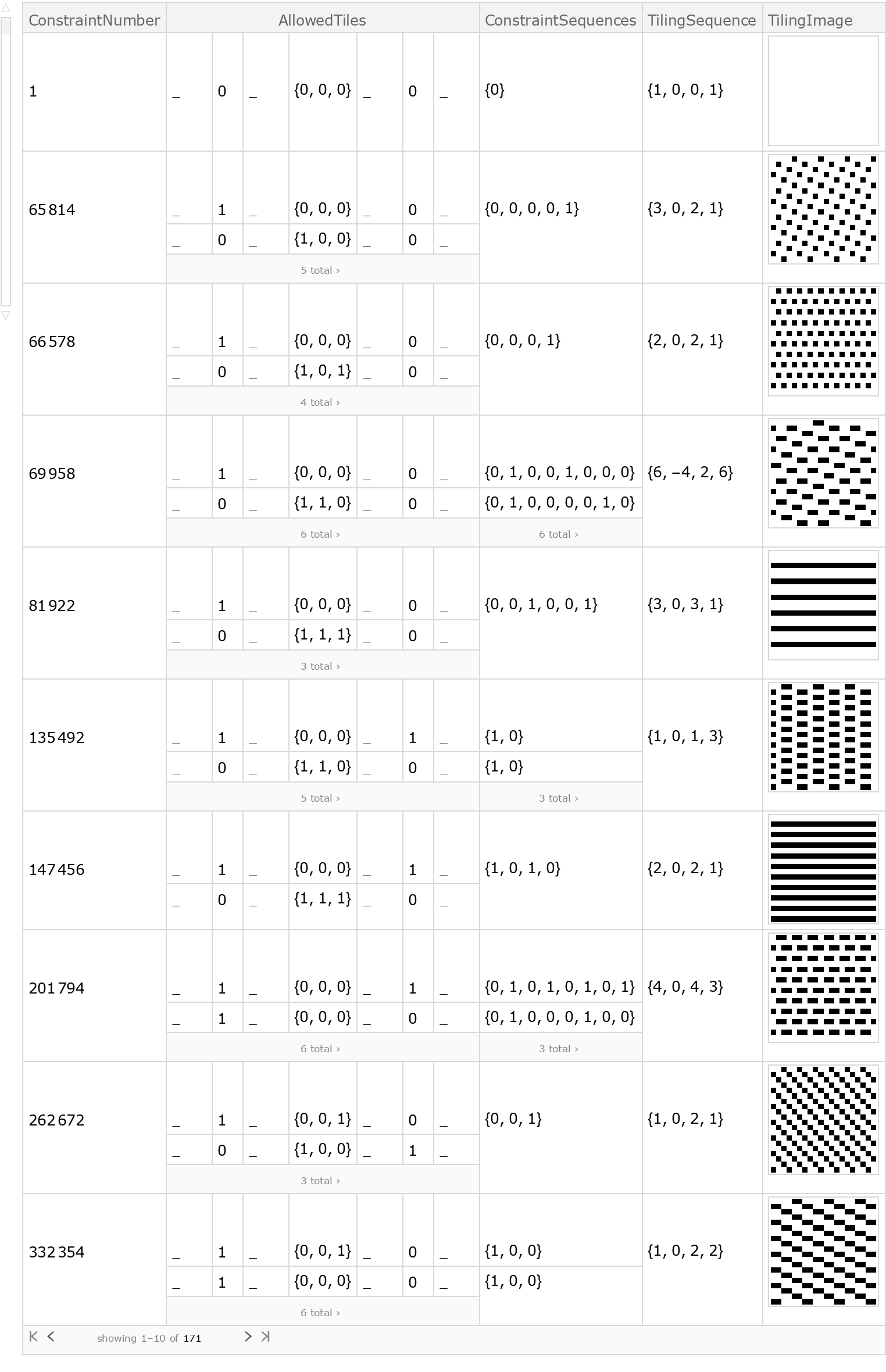Choose 3 tilings at random:

 In:=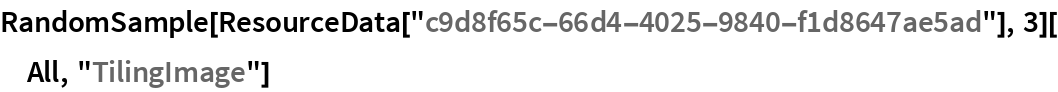Out=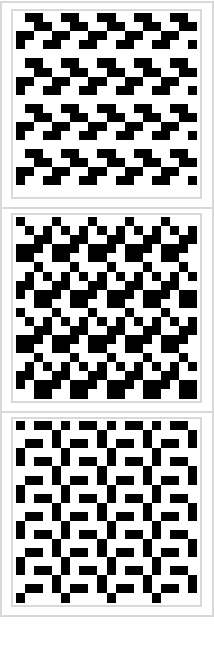Find the tilings with the most black cells:

 In:=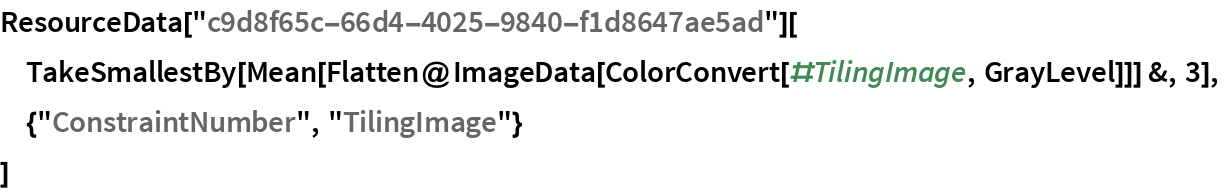Out=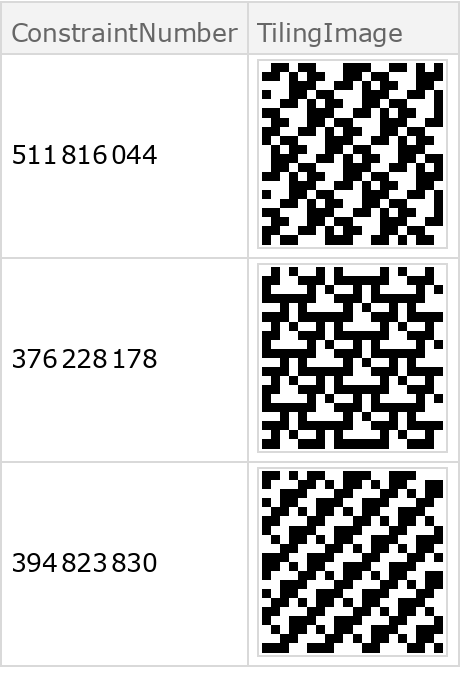In:=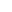Wolfram Research, "Minimal Inequivalent Square Tilings" from the Wolfram Data Repository (2017) https://doi.org/10.24097/wolfram.00988.data